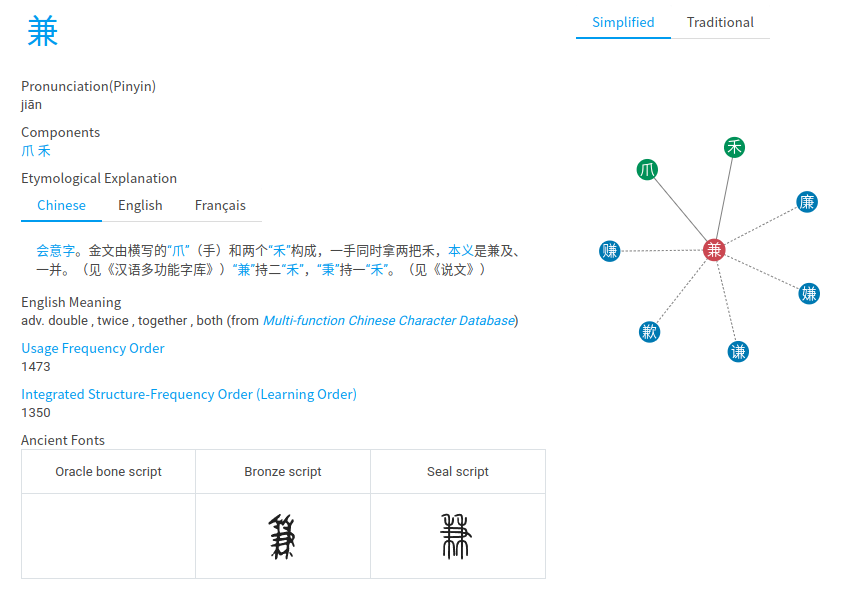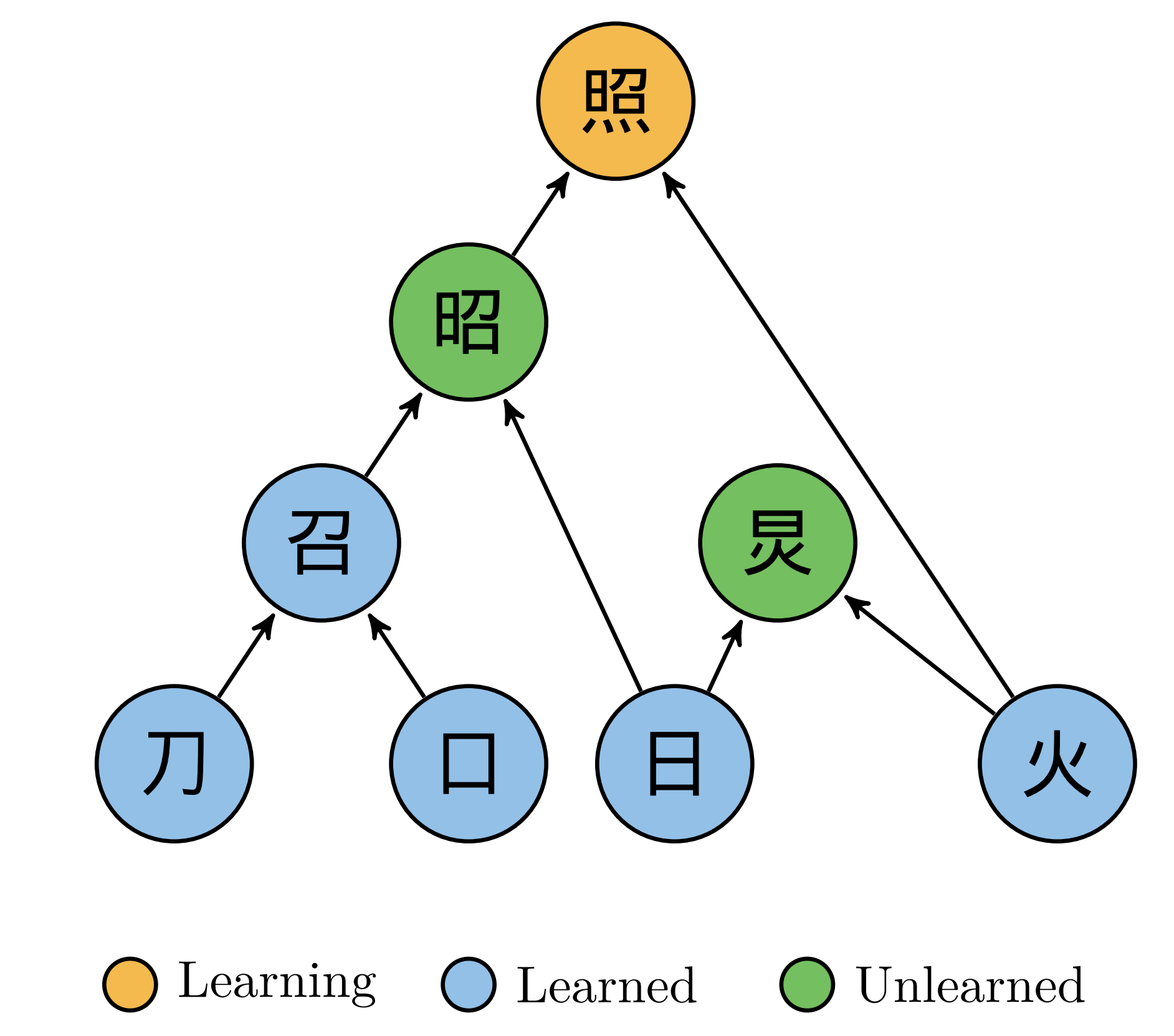# 汉字研究的工作被BBCFuture专栏述评

http://www.bbc.com/future/story/20130315-a-better-way-to-learn-chinese。述评写的非常好，比我们的文章的Introduction还要好。Philip Ball是牛人一名（Critical Mass等书的作者）。

### 研究问题### 数学模型\begin{align}
\tilde{W}^{i}= W^{i} + \sum_{j}\frac{a_{i}^{j}}{\sum_{k}a_{i}^{k}}\tilde{W}^{j}.
\end{align}

\begin{align}
\tilde{W}= W+ B\tilde{W} \Rightarrow \tilde{W}= \left(1-B\right)^{-1}W.
\end{align}